Tamilnadu State Board New Syllabus Samacheer Kalvi 12th Business Maths Guide Pdf Chapter 2 Integral Calculus I Ex 2.8 Text Book Back Questions and Answers, Notes.

## Tamilnadu Samacheer Kalvi 12th Business Maths Solutions Chapter 2 Integral Calculus I Ex 2.8

Using second fundamental theorem, evaluate the following:

Question 1.
$$\int_{0}^{1}$$ e2x dx
Solution: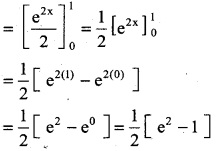Question 2.
$$\int_{0}^{1/4}$$ $$\sqrt { 1 -4x}$$ dx
Solution:Question 3.
$$\int_{0}^{1}$$ $$\frac { xdx }{x^2+1}$$
Solution: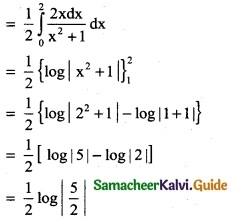Question 4.
$$\int_{0}^{3}$$ $$\frac { e^xdx }{1+e^x}$$
Solution:
$$\int_{0}^{3}$$ $$\frac { e^xdx }{1+e^x}$$
= {log |1 + ex|}$$_{0}^{3}$$
= log |1 + e³| – log |1 + e°|
= log |1 + e³| – log |1 + 1|
= log |1 + e³| – log |2|
= log |$$\frac { 1+e^3 }{2}$$|

Question 5.
$$\int_{0}^{1}$$ xe dx
Solution: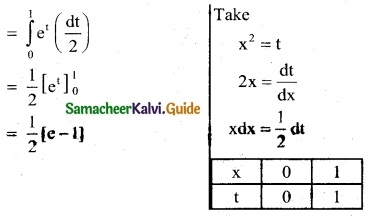Question 6.
$$\int_{1}^{e}$$ $$\frac { dx }{x(1+logx)^3}$$
Solution: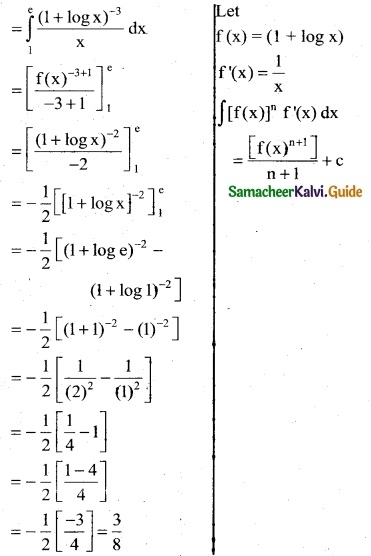Question 7.
$$\int_{-1}^{1}$$ $$\frac { 2x+3 }{x^2+3x+7}$$ dx
Solution: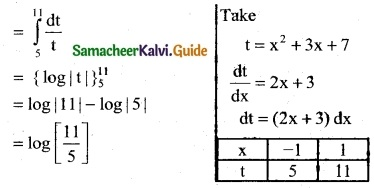Question 8.
$$\int_{0}^{π/2}$$ $$\sqrt { 1 +cosx}$$ dx
Solution:Question 9.
$$\int_{1}^{2}$$ $$\frac { x-1 }{x^2}$$ dx
Solution: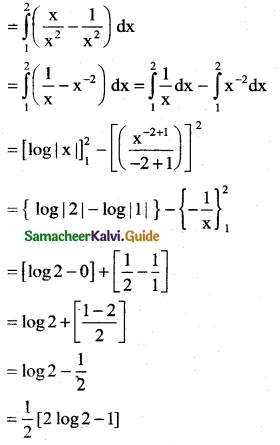Evaluate the following

Question 10.
$$\int_{1}^{4}$$ f(x) dx where f(x) = $$\left\{\begin{array}{l} 4 x+3,1 \leq x \leq 2 \\ 3 x+5,2 \end{array}\right.$$
Solution:
$$\int_{1}^{4}$$ f(x) dx
= $$\int_{1}^{2}$$ f(x) dx + $$\int_{2}^{4}$$ f(x) dx
= $$\int_{1}^{2}$$ (4x + 3) dx + $$\int_{2}^{4}$$ (3x + 5) dx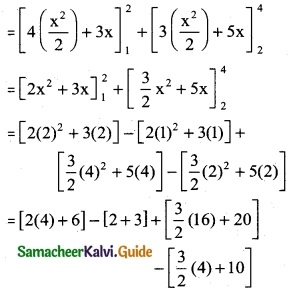(8 + 6) –  + [24 + 20] – [6 + 10]
= 14 – 5 + 44 – 16
= 58 – 21
= 37Question 11.
$$\int_{0}^{2}$$ f(x) dx where f(x) = $$\left\{\begin{array}{ll} 3-2 x-x^{2}, & x \leq 1 \\ x^{2}+2 x-3, & 1<x \leq 2 \end{array}\right.$$
Solution:
$$\int_{0}^{2}$$ f(x) dx
= $$\int_{0}^{1}$$ f(x) dx + $$\int_{1}^{2}$$ f(x) dx
= $$\int_{0}^{1}$$ (3 – 2x – x²) dx + $$\int_{1}^{2}$$ (x² + 2x – 3) dx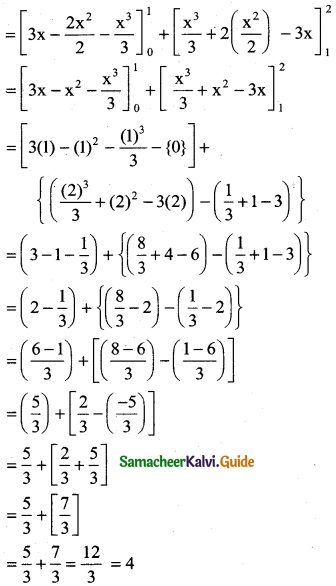Question 12.
$$\int_{-1}^{1}$$ f(x) dx where f(x) = $$\left\{\begin{array}{ll} x, & x \geq 0 \\ -x, & x<0 \end{array}\right.$$
Solution:
$$\int_{-1}^{1}$$ f(x) dx
$$\int_{-1}^{0}$$ f(x) dx + $$\int_{0}^{1}$$ f(x) dx
= $$\int_{-1}^{0}$$ (-x) dx + $$\int_{0}^{1}$$ x dx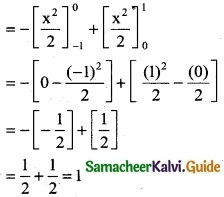Question 13.
f(x) = $$\left\{\begin{array}{l} c x, \quad 0<x<1 \\ 0, \text { otherwise } \end{array}\right.$$ find ‘c’ if $$\int_{0}^{1}$$ f(x) dx = 2
Solution:
Given
f(x) = $$\left\{\begin{array}{l} c x, \quad 0<x<1 \\ 0, \text { otherwise } \end{array}\right.$$
⇒ $$\int_{0}^{1}$$ f(x) dx = 2
⇒ $$\int_{0}^{1}$$ cx dx = 2
c[ $$\frac { x^2 }{ 2 }$$ ]$$_{0}^{1}$$ = 2
c[ $$\frac { 1 }{ 2 }$$ – 0 ] = 2
$$\frac { 1 }{ 2 }$$ = 2
⇒ c = 4